# Why more physics can help achieving better mathematics

## Abstract

In this paper, we discuss the question whether a physical “simplification” of a model makes it always easier to study, at least from a mathematical and numerical point of view. To this end, we give different examples showing that these simplifications often lead to worse mathematical properties of the solution to the model. This may affect the existence and uniqueness of solutions as well as their numerical approximability and other qualitative properties. In the first part, we consider examples where the addition of a higher-order term or stochastic noise leads to better mathematical results, whereas in the second part, we focus on examples showing that also nonlocal models can often be seen as physically more exact models as they have a close connection to higher-order models.

## Introduction

Differential equations are nowadays a standard tool to describe many physical phenomena and processes. The goal of this paper is to illustrate that, in many cases, a physical “simplification” of a model (meaning here the omission of higher-order derivatives or stochastic noise) does not imply a mathematical simplification as it reduces the mathematical “quality” of solutions in terms of regularity, uniqueness, numerical approximability and other properties such as, for example, long-term behaviour. This raises the question if it might be more adequate to study the physically more exact model with respect to mathematics and numerics. Besides, we also wish to draw attention to the less common nonlocal models: In many cases, the equation with higher-order terms can be transformed into a nonlocal equation, or it is at least an approximation, in some sense, of a nonlocal equation. In this way, nonlocal models can be seen as models comprising different local models as well as models of different characteristic scale.Footnote 1 This connection might also help improving the numerics of nonlocal equations, for which up to now less is known in comparison to the numerics of local models.

In the first part of this paper, we give a short introduction to some mathematical solution concepts for differential equation problems to make the following sections of this work easier to understand.

In the second part, we present some examples where the simplified model has a mathematically “worse” solution than the so-called regularised problem including higher-order terms or stochastic perturbations, which both have a physical meaning in most cases. We give a concrete example where the higher-order term already appears in the physical derivation of the model but is then omitted because it seems to be of negligibly small order of magnitude.

In the third and last part, we give some examples showing interesting connections between nonlocal models and local higher-order models, for example the transformation of a higher-order equation into a nonlocal one, or the approximation of a nonlocal equation via a higher-order equation.

## Different solution concepts

We briefly introduce some of the most important mathematical solution concepts for differential equation problems. Throughout this paper, let $$\varOmega$$ be an open subset of $$\mathbb {R}^d$$ with sufficiently smooth boundary. We start with the probably most popular concept, the concept of classical solutions: a classical solution is sufficiently often continuously differentiable and the differential equation is fulfilled pointwise everywhere. A similar concept is the concept of strong solutionsFootnote 2: a strong solution is sufficiently often differentiable in the weak sense and the equation is fulfilled pointwise almost everywhere. We call a function $$u:\varOmega \rightarrow \mathbb {R}$$ weakly differentiable in the direction $$x_i$$, $$i\in \{1,\ldots ,d\}$$, if it, together with its weak derivative $$v:\varOmega \rightarrow \mathbb {R}$$, fulfils some kind of integration-by-parts condition, i.e., the equation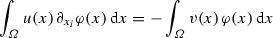(1)

holds for all test functions $$\varphi :\varOmega \rightarrow \mathbb {R}$$ that are infinitely many times continuously differentiable and vanish outside of a compact subset of the domain $$\varOmega$$. This concept of weak derivatives can be seen as a generalisation of the concept of classical derivatives since every integrable classical derivative fulfils Eq. (1). The boundary term appearing if integration by parts is applied vanishes here because the function $$\varphi$$ vanishes at the boundary of $$\varOmega$$.

The weak derivative also motivates another quite popular concept of solutions, the so-called weak solutions. One of the first using it was Jean Leray  in 1934 to prove the existence of solutions to the Navier–Stokes equations in two and three spatial dimensions. Since then it has become one of the most often used concepts of solutions. To obtain the weak formulation of the differential equation problem, we multiply the original differential equation with test functions $$\varphi$$ with the same properties as in the definition of the weak derivative. Then we integrate on both sides of the equation over $$\varOmega$$ and formally perform an integration by parts in the terms with higher derivatives such that half of the derivatives is shifted onto the test function $$\varphi$$. The solution to the resulting integral equation in an appropriate solution space we call weak solution. Note that the weak solution in general has a lower regularity than both classical and strong solutions, e.g., a weak solution to the common heat equation

\begin{aligned} \partial _t u + \alpha \; \varDelta u = f \end{aligned}
(2)

only has to be once differentiable in space in the weak sense although two derivatives appear in the original equation. It can be shown that a classical solution is also always a weak solution. Therefore, the concept of weak solutions is a generalisation of the concept of classical solutions. It allows for example discontinuities in some derivatives of the solution and is able to handle more general data. Moreover, there are many (especially nonlinear) problems that do not admit classical solutions but weak solutions. A more detailed introduction into the concept of weak solutions can, e.g., be found in Chipot  or Roubíček .

The last concept of solutions we want to discuss in detail is the concept of the so-called (Young-) measure-valued solutions. In the case of a nonlinear equation, even the existence of weak solutions sometimes cannot be proven, especially if certain oscillation effects come into play. These oscillation effects are now covered by a measure-valued mapping $$\nu$$ which replaces the function u (or derivatives of u, respectively) in the weak formulation in the term with the nonlinearity. Thus, a measure-valued solution always consists of the function u and the measure-valued mapping $$\nu$$. The concept of measure-valued solutions is again a generalisation of the concept of weak solutions, since every weak solution is also a measure-valued solution taking as $$\nu$$ the point measure of the solution (or derivatives of it, respectively). However, the concept of measure-valued solutions is quite controversial, as it is a rather weak concept. For example, uniqueness of measure-valued solutions cannot be expected in general. Even for problems that admit a unique weak solution, existence of infinitely many measure-valued solutions can be shown . Apart from that, the numerical approximability is worse than for weak solutions, which, because of their nice structure, can be easily approximated via the finite element method, for example. A more detailed introduction into the concept of measure-valued solutions can, e.g., be found in Málek et al. .

Between these concepts there are many others, for example very weak solutions where, in contrast to weak solutions, all derivatives are shifted onto the test function, or entropy solutions which have to fulfil an additional entropy inequality. An overview of some of these concepts using the example of the Navier–Stokes equations can be found in Amann . Another overview of results concerning classical, weak and measure-valued solutions in the theory of elastodynamics is given in Emmrich and Puhst .

## Higher-order terms in physics and mathematics

In this section, we present two examples where physically motivated higher-order terms lead to mathematically “better” solutions to the regularised problem than to the original problem. Additionally, we consider the regularisation using stochastic perturbations instead of higher-order terms, which requires less assumptions on the given data, compared to the problem without a stochastic perturbation, to obtain existence and uniqueness of solutions.

### Example 1: backward–forward heat equation

The first example is the so-called backward–forward heat equation

\begin{aligned} \partial _t u - \nabla \cdot \phi (\nabla u)=0, \end{aligned}
(3)

where u represents the temperature and $$\phi$$ is a nonmonotone function representing the heat flux density. This equation occurs, for example, in cases where Fourier’s law of heat conduction cannot be applied to simplify the general heat equation. Apart from thermodynamics, it is also very important for the so-called anisotropic diffusion considered in image processing.

There are several articles proving existence of measure-valued solutions to the backward-forward heat equation, for example, Slemrod  and Thanh et al. . In both articles, the method of regularisation is used to prove existence. In the first work , the term $$\varepsilon \; \varDelta ^2 u$$ is added to the left-hand side, where $$\varepsilon >0$$ is small. For the regularised equation

\begin{aligned} \partial _t u_\varepsilon - \nabla \cdot \phi (\nabla u_\varepsilon ) + \varepsilon \; \varDelta ^2 u_\varepsilon = 0, \end{aligned}
(4)

existence of weak solutions can be proven. The limit $$\varepsilon \rightarrow 0$$ then yields the existence of a measure-valued solution to Eq. (3). As mentioned in Sect. 2 on solution concepts, measure-valued solutions are weaker than weak solutions. Thus, we get “better” solutions to the regularised equation with a higher-order term than to the original equation without this higher-order term.

In the second work , the term $$-\,\varepsilon \;\varDelta \partial _t u$$ is added to the left-hand side of the backward–forward heat equation. Again, existence of weak solutions can be shown for the regularised equation

\begin{aligned} \partial _t u_\varepsilon -\nabla \cdot \phi (\nabla u_\varepsilon ) - \varepsilon \;\varDelta \partial _t u_\varepsilon =0, \end{aligned}
(5)

but in the limit $$\varepsilon \rightarrow 0$$, we again only end up with a measure-valued solution to the original equation.

So far, the existence of weak solutions to the backward–forward heat equation is only known in a special case, where the spatial dimension is equal to one and where the heat flux density $$\phi$$ is piecewise linear (cf. [12, 13]). Besides, uniqueness of solutions is also a problem. Uniqueness of measure-valued solutions cannot be expected due to the solution concept, and in the case mentioned above, where existence of weak solutions is known, it can additionally be proven that there exist infinitely many weak solutions. So far, uniqueness is only known for a special kind of classical solutions and again only in the case of one spatial dimension and for special cases of the heat flux density $$\phi$$ (cf. [14, 15]). Whether solutions of this kind do even exist, could, to the best knowledge of the authors, not yet be proven.

These observations raise one central question of this article: Is passing to the limit $$\varepsilon \rightarrow 0$$ and thus reducing the mathematical quality of solutions necessary for the equations to be an adequate model of the underlying physics? In fact, both regularisations shown above are motivated by physics. Slemrod  refers to the higher-order theory of heat conduction due to Maxwell , which is also mentioned in Truesdell and Noll . It is based upon a moment expansion of the Boltzmann equation, which leads to an infinite hierarchy of coupled moment equations for the mass density, the momentum density, the energy density, the energy flux etc., and has to be truncated by appropriate closure approximations. The common approximation of the heat flux by Fourier’s law in the mean energy balance equation gives the standard heat conduction equation. However, already in  it was shown for rarified gases that higher-order terms can arise due to density gradient contributions to the energy balance, and in  it was pointed out that a double Laplacian similar to Eq. (4) also occurs in the Cahn–Hilliard equation which describes the process of phase separation in a two-component binary fluid. Moment expansions of the Boltzmann equation, resulting in hydrodynamic balance equations for charge carrier densities and electron temperature, have also widely been used in nonlinear electron transport in semiconductors [18,19,20], giving higher-order terms at various levels of approximation.

Thanh et al.  describe the regularisation term as some kind of viscous effects referring, amongst others, to Binder et al.  and Novick-Cohen and Pego .

Indeed, there is a whole mathematical theory called vanishing viscosity method based upon the regularisation with a diffusive term multiplied with the regularisation parameter $$\varepsilon$$. At the end, letting the parameter $$\varepsilon$$ tend to zero yields a solution to the original problem. The method goes back to von Neumann and Richtmyer  who introduced it as a method to calculate hydrodynamic shocks numerically.

One of the simplest examples for which the vanishing viscosity method can successfully be applied is the famous Burgers’ equation

\begin{aligned} \partial _t u + u \;\partial _x u =0, \end{aligned}
(6)

which can be seen as a simple model for a one-dimensional flow. To apply the vanishing viscosity method, the diffusion term $$\varepsilon \; \partial _{xx}u$$ is added,

\begin{aligned} \partial _t u + u \;\partial _x u -\varepsilon \; \partial _{xx}u=0, \end{aligned}
(7)

where $$\varepsilon$$ is the regularisation parameter mentioned above. Intuitively, the discontinuities that may appear in the case of the inviscid Burgers’ equation (6) are first smoothed out by the additional diffusion term and then, this smoothing effect is reduced more and more (cf. Fig. 1), such that at the end, a solution to the original Eq. (6) is obtained. A detailed discussion of the application of the vanishing viscosity method in the case of the Burgers’ equation can, e.g., be found in the lecture notes of Kruzhkov on first-order quasilinear partial differential equations .

Another example where the vanishing viscosity method can successfully be applied is a system of Boussinesq equations, shown in the monograph of Guo et al. . It reads

\begin{aligned} \begin{aligned}&\partial _t \rho + \alpha \; \partial _x u + \beta \; \partial _x (u\rho ) =0, \\&\partial _t u + \gamma \;\partial _x \rho + \delta \; u\; \partial _x u - \nu \; \partial _{xxt} u =0, \end{aligned} \end{aligned}
(8)

and describes the propagation of the long surface wave in a pipe with constant depth. Here, $$\rho$$ is the density, u is the velocity, $$\omega =1+\delta \rho$$ denotes the altitude from the bottom to the free surface of the flow and $$\alpha ,\beta ,\gamma ,\delta , \nu$$ are constants. In this case, the regularisation term $$\varepsilon \; \partial _{xx} \rho$$ is added to the first equation and at the end, $$\varepsilon$$ tends to zero. Here, the regularised problem admits a unique classical smooth solution, whereas the original problem only admits weak solutions.

A broad overview of other examples for the vanishing viscosity method can also be found in Guo et al. .

### Example 2: kinetic models for dilute polymers

In other examples, like the following one, the regularisation term is already existent in the derivation of the physical model but is then omitted because it seems to be of negligibly small order of magnitude. Barrett and Süli  consider a kinetic bead-spring model for dilute polymers, where the extra-stress tensor is defined through the associated probability density function $$\psi$$. This function satisfies the Fokker–Planck-type parabolic equation

\begin{aligned}&\partial _t \psi + \left( u\cdot \nabla _x\right) \psi + \nabla _q\cdot \left( \left( \nabla _x\; \mathscr {J}^x_{l_0,q}\; u \right) q\; \psi \right) \nonumber \\&\quad = \varepsilon \; \varDelta _x \psi + \frac{1}{2\lambda }\nabla _q \cdot \left( \nabla _q\; \psi + U'\;q\; \psi \right) . \end{aligned}
(9)

Here, u is the velocity of the fluid considered, q is the elongation vector of the dumbbell representing a polymer chain, $$\mathscr {J}^x_{l_0,q}$$ is the directional Friedrichs mollifier with respect to x over an interval of length $$l_0\vert q\vert$$ in the direction q, and U is the potential of the elastic force of the spring connecting two beads. The constant $$\varepsilon$$ corresponds to the quantity $$\frac{\text {De}}{\text {Pe}}$$, where $$\text {De}$$ denotes the Deborah number and $$\text {Pe}$$ the Péclet number, and the constant $$\lambda$$ corresponds to the relaxation time constant of the dumbbells.

One interesting feature of this model is the presence of the diffusion term $$\varepsilon \;\varDelta _x\;\psi$$ on the right-hand side of Eq. (9). As Barrett and Süli  already mention, this term is usually omitted in standard derivations of bead-spring models, because it is several orders of magnitude smaller than the other terms in Eq. (9). Actually, Bhave et al.  estimate the quantity $$\frac{De}{Pe}$$ to be in the range of $$10^{-9}$$$$10^{-7}$$, whereas the expected important length scales of stress diffusion start at $$10^{-5}$$$$10^{-3}$$.

Mathematically however, omitting the diffusion term is quite detrimental as it leads to a hyperbolically degenerate parabolic equation which is much harder to handle than Eq. (9).Footnote 3 In fact, the existence result in the case $$\varepsilon =0$$ is again proven via showing the existence of solutions for $$\varepsilon >0$$ and then passing to the limit as $$\varepsilon \rightarrow 0$$. This leads to less regularity for the probability density function $$\psi$$. So again we state that the original model with the higher-order term delivers mathematically “better” solutions than the model without this higher-order term. In this case, the model with the higher-order term even seems to be more adequate physically.

### Example 3: regularisation by noise

A quite recent approach to regularise an equation is to add a certain stochastic noise in order to obtain the existence of a unique solution where, without noise, only existence or uniqueness or none of these two is known so far.

There is some work by, e.g., Gyöngy and Pardoux [30, 31] using additive noise to prove existence of a unique solution under assumptions which, in the deterministic case, are so far not known to suffice for obtaining existence or uniqueness. Gyöngy and Pardoux [30, 31] consider the equation

\begin{aligned} \partial _t u(x,t) - \partial _{xx} u(x,t) =f(x,t,u(x,t)) + \partial _{tx} W(x,t) \end{aligned}
(10)

equipped with either homogeneous Dirichlet or homogeneous Neumann boundary conditions, where f is a nonlinear function and $$\partial _{tx} W$$ denotes space-time white noise. Under the assumption that f satisfies some measurability and boundedness condition, Gyöngy and Pardoux [30, 31] are able to prove existence and uniqueness of a solution in a generalized sense defined in the work of Walsh , which may be compared to a very weak solution (cf. Sect. 2) but in a stochastic sense. In the deterministic case, the assumptions on f are, to the best knowledge of the authors, not enough to prove existence or uniqueness of solutions.

More recently, there has been research on linear multiplicative noise by, e.g., Flandoli et al. , considering the linear transport equation

\begin{aligned} \partial _t u + b\cdot \nabla u = 0 \end{aligned}
(11)

driven by the vector field b. Assuming that b is sufficiently regular, uniqueness of solutions to the initial-boundary value problem governed by this equation can be proven (cf., e.g., DiPerna and Lions  or Ambrosio ), but if this is not the case then examples of non-uniqueness are known, as is shown in the work of Flandoli et al. . However, if a certain amount of linear multiplicative noise is added to Eq. (11), existence and uniqueness of solutions can be proven under weaker assumptions on b, see again . To be precise, the stochastic equation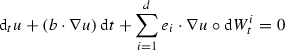(12)

is considered, where $$e_i$$, $$i=1,\ldots ,d$$, are the unit vectors in $$\mathbb {R}^d$$,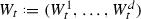is a standard Brownian motion in $$\mathbb {R}^d$$, and the notation $$\circ$$ is used for the stochastic integration in the sense of Stratonovich.

Since real-world systems often include noise, the consideration of stochastic differential equations is physically also very important, and there are many works considering the influence of noise on various physical systems. We just want to mention some examples here. Additive noise has been shown to have an important effect, e.g., upon chimera states (coexisting coherent and incoherent space-time patterns in networks), which can be either destructive, see, e.g., Loos et al. [36, 37], or constructive, see, e.g., Zakharova et al. [38, 39]. Multiplicative noise has been considered, for example, in the work on nonequilibrium phase transitions by Van den Broeck et al. .

## Connection of higher-order and nonlocal equations

In this part, we show some interesting connections between nonlocal models and local higher-order models. As it turns out, many equations containing higher-order terms can be rewritten as some nonlocal equation or can be seen as approximations of nonlocal equations. Thus, nonlocal models can also be seen as physically more exact regularisations of local models.

### Green’s function as integral kernel

Let us start with a simple example, which was, amongst others, mentioned in Duruk et al. [41,42,43]. We consider the equation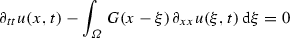(13)

arising, e.g., in the nonlocal theory of elasticity which considers the stress at a point x not only as a function of the strain at the point x but the strain field at every point in the body. Thus, it also takes long-range interactions between the particles into account. In this case, u represents the displacement field and G is the kernel function representing the stress-strain relation. Here, G is the Green’s function for the differential operator $$1-\varepsilon \;\partial _{xx}$$ considered on the full space (therefore we can neglect boundary conditions), i.e.,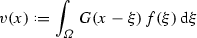(14)

is the unique solution to the problem

\begin{aligned} v(x) - \varepsilon \;\partial _{xx} v(x) = f(x). \end{aligned}
(15)

Based on Eq. (13), we can now conclude that $$\partial _{tt} u$$ at least formally fulfils

\begin{aligned} \partial _{tt} u(x,t) - \varepsilon \;\partial _{xx} \partial _{tt} u(x,t) = \partial _{xx} u(x,t). \end{aligned}
(16)

Therefore, we can see the nonlocal Eq. (13) as a regularised form of the usual wave equation

\begin{aligned} \partial _{tt} u(x,t) - \partial _{xx} u(x,t)=0. \end{aligned}
(17)

This method can obviously be applied using any arbitrary Green’s function G in an arbitrarily high spatial dimension, generating many different regularisations of arbitrarily high order. Considering, for example, the general differential operator(18)

where $$n\in \mathbb {N}$$, $$a_k>0$$, $$k=1,\ldots ,n$$, the Green’s function for A reads

\begin{aligned} G(x)= \mathscr {F}^{-1}\left( \left( 1+\sum _{k=1}^n a_k \vert \omega \vert ^{2k}\right) ^{-1}\right) , \end{aligned}
(19)

where $$\mathscr {F}^{-1}$$ denotes the inverse Fourier transform. If we start, for example, with a nonlocal equation, this operator A can be used to approximate the differential operator of which the given kernel function is the Green’s function. This has been done, for example, for lattice models in nonlocal continuum mechanics in Eringen [44, Ch. 6.9] and Lazar et al. . Thus, we can consider nonlocal models as a generalisation of local ones as different kernels lead to different local, potentially higher-order models.

### Nonlocal coupling in reaction-diffusion systems

A more involved example is a reaction-diffusion system with asymmetric nonlocal coupling, studied in Siebert et al. , which is derived as a limiting case of the activator-inhibitor reaction-diffusion-advection model

\begin{aligned} \partial _t u(x,t)= & {} F(u(x,t)) - g\;w(x,t) + \partial _{xx} u(x,t),\nonumber \\ \tau \partial _t w(x,t)= & {} h\;u(x,t) - f\; w(x,t)+ \xi \; \partial _x w(x,t)\nonumber \\&\quad +\,D\; \partial _{xx} w(x,t). \end{aligned}
(20)

Here, u and w are the activator and the inhibitor, respectively, linearly coupled by the terms $$-\,g\;w$$ and $$h\;u$$ with $$g,h\in \mathbb {R}$$, F is a nonlinear function, $$\tau$$ is the inhibitor relaxation time, f is a constant, $$\xi$$ represents the advection velocity and $$D>0$$ is the inhibitor diffusion coefficient. Passing to the limit $$\tau \rightarrow 0$$, i.e., the case of fast inhibitor dynamics, the Green’s function is used to reformulate the system by writing the solution w of the second equation of (20) as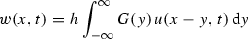(21)

with the Green’s function G defined as

\begin{aligned} G(x) = \frac{f}{\sqrt{\xi ^2 + 4Df}} e^{-\left( \sqrt{\xi ^2 + 4Df}/2D\right) \;\vert x\vert } e^{\xi x/2D}. \end{aligned}
(22)

Inserting Eq. (21) into the first equation of (20), the reaction-diffusion equation with asymmetric nonlocal coupling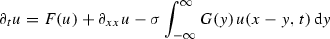(23)

is obtained, where $$\sigma = g\;h$$ denotes the nonlocal coupling strength.

This method is more or less the inverse of the method shown in Sect. 4.1. If we solve the first equation of (20) for w and insert it into the second equation, we end up with the same local higher-order equation which we would get using the method of Sect. 4.1. Thus, higher-order and nonlocal terms may be considered as physically representing some kind of nonlocal coupling.

### Expansion of integral operators

Another way to approximate a nonlocal equation by a local one including higher-order terms is the expansion of the corresponding integral operator. We will illustrate this by the example of a linear peridynamic model,Footnote 4 as was done in Emmrich and Weckner . We consider the equation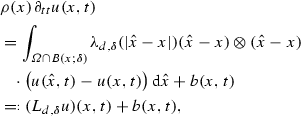(24)

where $$\rho$$ denotes the mass density, and u is the displacement field of the body, $$\varOmega$$ represents the volume that the body occupies, $$B(x,\delta )$$ is the ball of radius $$\delta$$ around x, d is the spatial dimension, $$\delta >0$$ is the so-called peridynamic horizon of interaction, $$\lambda _{d,\delta }$$ is a real-valued function which depends upon d and $$\delta$$ and determines the specific material model, and b represents the external forces. The symbol $$\otimes$$ denotes the outer product. Additionally, the function $$\lambda _{d,\delta }$$ has the property

\begin{aligned} \lambda _{d, \delta }(r) = 0\quad \text {for all }\quad r \ge \delta . \end{aligned}
(25)

We now consider only the integral operator $$L_{d,\delta }$$. The idea is to perform a Taylor expansion of the argument u up to the order of m, supposing sufficient regularity. In order to make the following easier to read, we omit the time dependence of u and obtain

\begin{aligned} u(\hat{x}) - u(x) = \sum _{k=1}^m \frac{1}{k!} \left( (\hat{x}-x)\cdot \nabla \right) ^k u(x) + r_m(u; \hat{x}, x)\qquad \end{aligned}
(26)

with some remainder $$r_m(u;\hat{x},x)$$ of order $$o (\vert \hat{x} - x \vert ^m)$$. Now we insert the Taylor expansion into the operator $$L_{d,\delta }$$ and get

\begin{aligned} \left( L_{d,\delta } u\right) (x) = \sum _{k=1}^m \left( L_{d,\delta }^{(k)} u\right) (x) + R_{m;d,\delta }(u;x) \end{aligned}
(27)

with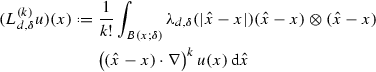(28)

and again some remainder $$R_{m;d,\delta }(u;x)$$ of order $$o(\delta ^{m-2})$$ in an appropriate norm (for details see Emmrich and Weckner [48, Theorem 3.2]). Since all derivatives appearing in the integrand of Eq. (28) only depend on x and not on $$\hat{x}$$, the operators $$L_{d,\delta }^{(k)}$$ are local differential operators of order k. Furthermore, for odd k, the integrand is an odd function in $$\hat{x}-x$$ so that the integral vanishes and thus $$(L_{d,\delta }^{(k)} u)(x) =0$$ for all odd k. In summary, we can approximate the nonlocal Eq. (24) by the local equation

\begin{aligned} \rho (x)\; \partial _{tt} u(x,t) = \sum _{k=1}^{[m/2]} (L_{d,\delta }^{(2k)} u)(x) + b(x,t). \end{aligned}
(29)

For the second order $$m=2$$, this approximation yields the classical Navier equation of linear elasticity

\begin{aligned} \rho (x)\; \partial _{tt} u(x,t) = \mu \varDelta u(x,t) +(\lambda +\mu ) \nabla (\nabla \cdot u(x,t))+ b(x,t) \end{aligned}
(30)

with the Lamé coefficients $$\mu =\lambda =3K/5$$ where K denotes the bulk modulus. Using the above approximation, it is shown that the nonlocal operator $$L_{d,\delta }$$ converges to the local operator L of the Navier equation for vanishing nonlocality $$\delta \rightarrow 0$$, at least in the interior of the domain $$\varOmega$$. Near the boundary, this is to the best knowledge of the authors not yet known. The reason for that is the problem of finding appropriate boundary conditions for the Navier equation. For the peridynamic model, there are no boundary conditions since no spatial derivatives appear. More information about vanishing nonlocality in linear and also nonlinear peridynamic models can be found, e.g., in Puhst .

A quite similar approach to the one above, the so-called inner expansion, was used by Arndt and Griebel  to derive a new scheme upscaling the atomistic level to the continuum level for the model of a crystalline solid. There, a Taylor expansion of the deformation function around the points of the discrete lattice representing the atoms is inserted into the local energy potential to obtain, after some more steps, a continuum formulation which represents the upscaled model. One of this technique’s advantages is the well-posedness of the resulting equation, which is not guaranteed by other techniques like the direct expansion technique proposed by Kruskal and Zabusky  and Zabusky and Kruskal , which relies on a Taylor expansion of the right-hand side of the equation instead of the deformation function. On the other hand, it covers higher-order effects to an arbitrarily high order in contrast to the common scaling technique, which, simply spoken, lets the number of atoms tend to infinity, and was studied, amongst others, by Blanc et al. . This inclusion of higher-order effects prevents the loss of regularity of the solution that is often observed for the scaling technique because the dispersion which the discrete system inherits is not contained in the resulting continuum system.

Again we see how important higher-order terms are and, although there is no nonlocal model involved in this example, there is still a connection to nonlocal models since they can also be seen as some kind of upscaling of a microscale model, as already mentioned in the introduction.

## Conclusion

In the first part of this paper, we presented many different examples showing that simplifications of differential equations in the physical sense often lead to worse mathematical properties of the solutions to these equations. This may affect the existence of various types of solutions, the uniqueness of these solutions if they exist, their numerical approximability, and their qualitative properties such as long-term behaviour. The example of the kinetic model for dilute polymers (Sect. 3.2), studied in Barrett and Süli , shows that the higher-order term—artificially added to the equation in order to regularise it— is in some cases already existent in the original physical model. However, it is usually omitted because its order of magnitude is several orders smaller than the other terms appearing in the model. This sheds new light on the question if physical simplification is admissible, or if, on the contrary, even small terms should be kept in physical models since they improve the numerical solvability and the mathematical properties of the solution.

In the second part, we extended our results by demonstrating an intriguing connection between nonlocal and local higher-order models. The example of Green’s function as the integral kernel of the nonlocal equation (Sect. 4.1) shows that many local higher-order equations can be rewritten as a nonlocal equation, and in this sense nonlocal models can be seen as a generalisation of local models. The example of the expansion of integral operators (Sect. 4.3) shows that even in more general situations, there is still a connection between nonlocal models and local higher-order models since the latter can be used as an approximation of the former. Overall, we wish to motivate the reader to consider also nonlocal models when studying a physical problem, especially in applications where nonlocal models have not received much attention previously. The connection mentioned might also improve the numerical understanding of nonlocal models.

As a physical interpretation of this connection, we suggest that nonlocal coupling terms can in certain situations be avoided by considering a more detailed description using additional dynamical variables. Considering a single differential equation with higher-order derivatives is equivalent to a system of several differential equations with lower-order derivatives, i.e., to extending the number of degrees of freedom of a dynamical model. These additional differential equations can be eliminated using Green’s functions, which results in nonlocal models with fewer dynamical variables. However, some nonlocal models like, for example, peridynamics would, at least formally, require an infinite number of degrees of freedom to be exactly represented. Additionally, they need much less regularity and are therefore more suitable to study discontinuous phenomena like, e.g., crack propagation.

1. Sometimes, nonlocal models can be seen as an upscaling of a local, microscale model, for example the theory of peridynamics . The purpose of peridynamic models is to be computationally faster than molecular dynamics but to preserve characteristic properties of molecular dynamics that are not recovered by classical continuum mechanics.

2. The term “strong” solution is not uniquely determined in the mathematical literature. Many authors use it as a synonym for classical solutions, but we will stick here with the definition given in Schweizer , since in this way, it is clearly separated from the other definitions.

3. If a parameter tends to zero and thus changes the type of the equation, this phenomenon is called singular limit. Nice overviews of such singular limits in hydrodynamics and thermodynamics of viscous fluids can be found in Masmoudi  and Feireisl and Novotný , respectively.

4. The peridynamic model goes back to Silling , who introduced it as an alternative to the classical elasticity model, replacing the local stress by a nonlocal one independent of the spatial gradients of deformation.

## References

1. Seleson P, Parks ML, Gunzburger M, Lehoucq RB (2009) Peridynamics as an upscaling of molecular dynamics. Multiscale Model Simul 8(1):204–227

2. Schweizer B (2013) Partielle differentialgleichungen. Springer, Berlin

3. Leray J (1934) Sur le mouvement d’un liquide visqueux emplissant l’espace. Acta Math 63(1):193–248

4. Chipot M (2009) Elliptic equations: an introductory course. Birkhäuser advanced texts: Basel textbooks. Birkhäuser Verlag, Basel

5. Roubíček T (2013) Nonlinear partial differential equations with applications. In: International Series of Numerical Mathematics, vol 153, 2nd edn. Springer, Basel

6. Roubíček T, Hoffmann KH (1995) About the concept of measure-valued solutions to distributed parameter systems. Math Methods Appl Sci 18(9):671–685

7. Málek J, Nečas J, Rokyta M, Růžička M (1996) Weak and measure-valued solutions to evolutionary PDEs. Applied mathematics and mathematical computation, vol 13. Chapman & Hall, London

8. Amann H (2000) On the strong solvability of the Navier–Stokes equations. J Math Fluid Mech 2(1):16–98

9. Emmrich E, Puhst D (2015) Survey of existence results in nonlinear peridynamics in comparison with local elastodynamics. Comput Methods Appl Math 15(4):483–496

10. Slemrod M (1991) Dynamics of measure valued solutions to a backward–forward heat equation. J Dyn Differ Equ 3(1):1–28

11. Thanh BLT, Smarrazzo F, Tesei A (2014) Sobolev regularization of a class of forward–backward parabolic equations. J Differ Equ 257(5):1403–1456

12. Höllig K (1983) Existence of infinitely many solutions for a forward backward heat equation. Trans Am Math Soc 278(1):299–316

13. Höllig K, Nohel JA (1983) A diffusion equation with a nonmonotone constitutive function. In: Systems of nonlinear partial differential equations (Oxford, 1982) NATO advanced science institutes series C: mathematical physics science, vol 111, Reidel, Dordrecht-Boston, pp 409–422

14. Lair AV (1985) Uniqueness for a forward backward diffusion equation. Trans Am Math Soc 291(1):311–317

15. Lair AV (1988) Uniqueness for a forward backward diffusion equation with smooth constitutive function. Appl Anal 29(3–4):177–189

16. Maxwell JC (1879) On stresses in rarified gases arising from inequalities of temperature. Philos Trans R Soc Lond 170:231–256

17. Truesdell C, Noll W (1965) The non-linear field theories of mechanics. In: Handbuch der Physik, Band III/3. Springer, Berlin, pp 1–602

18. Quade W, Rudan M, Schöll E (1991) Hydrodynamic simulation of impact ionization effects in p-n junctions. IEEE Trans CAD 10(10):1287–1294

19. Quade W, Schöll E, Rudan M (1993) Impact ionization within the hydrodynamic approach to semiconductor transport. Solid State Electron 36(10):1493–1505

20. Schöll E (1998) Theory of transport properties of semiconductor nanostructures. Chapman and Hall, London

21. Binder K, Frisch HL, Jäckle J (1986) Kinetics of phase separation in the presence of slowly relaxing structural variables. J Chem Phys 85(3):1505–1512

22. Novick-Cohen A, Pego RL (1991) Stable patterns in a viscous diffusion equation. Trans Am Math Soc 324(1):331–351

23. von Neumann J, Richtmyer RD (1950) A method for the numerical calculation of hydrodynamic shocks. J Appl Phys 21:232–237

24. Chechkin GA, Goritsky AY (2009) S. N. Kruzhkov’s lectures on first-order quasilinear PDEs. In: Analytical and numerical aspects of partial differential equations, Walter de Gruyter, Berlin, pp 1–67 (translated from the Russian by Boris P. Andreianov)

25. Guo B, Bian D, Li F, Xi X (2017) Vanishing viscosity method: solutions to nonlinear systems. De Gruyter, Berlin

26. Barrett JW, Süli E (2007) Existence of global weak solutions to some regularized kinetic models for dilute polymers. Multiscale Model Simul 6(2):506–546

27. Bhave AV, Armstrong RC, Brown RA (1991) Kinetic theory and rheology of dilute, nonhomogeneous polymer solutions. J Chem Phys 95(4):2988–3000

28. Masmoudi N (2007) Examples of singular limits in hydrodynamics. In: Handbook of differential equations: evolutionary equations, vol III. Elsevier, Amsterdam, pp 195–275

29. Feireisl E, Novotný A (2009) Singular limits in thermodynamics of viscous fluids. Advances in mathematical fluid mechanics. Birkhäuser, Basel

30. Gyöngy I, Pardoux É (1993) On quasi-linear stochastic partial differential equations. Probab Theory Relat Fields 94(4):413–425

31. Gyöngy I, Pardoux É (1993) On the regularization effect of space-time white noise on quasi-linear parabolic partial differential equations. Probab Theory Relat Fields 97(1–2):211–229

32. Walsh JB (1986) An introduction to stochastic partial differential equations. In: École d’été de probabilités de Saint-Flour, XIV–1984 (Lecture Notes in Mathematics), vol 1180. Springer, Berlin, pp 265–439

33. Flandoli F, Gubinelli M, Priola E (2010) Well-posedness of the transport equation by stochastic perturbation. Invent Math 180(1):1–53

34. DiPerna RJ, Lions PL (1989) Ordinary differential equations, transport theory and Sobolev spaces. Invent Math 98(3):511–547

35. Ambrosio L (2004) Transport equation and Cauchy problem for $$BV$$ vector fields. Invent Math 158(2):227–260

36. Loos S, Claussen JC, Schöll E, Zakharova A (2016) Chimera patterns under the impact of noise. Phys Rev E 93(012):209

37. Zakharova A, Loos SAM, Siebert J, Gjurchinovski A, Claussen JC, Schöll E (2016) Controlling chimera patterns in networks: interplay of structure, noise, and delay. In: Schöll E, Klapp SHL, Hövel P (eds) Control of self-organizing nonlinear systems. Springer, Berlin, pp 3–23

38. Semenova N, Zakharova A, Anishchenko V, Schöll E (2016) Coherence-resonance chimeras in a network of excitable elements. Phys Rev Lett 117(014):102

39. Zakharova A, Semenova N, Anishchenko VS, Schöll E (2017) Noise-induced chimera states in a neural network. In: Springer proceedings in mathematics and statistics. arXiv:1611.03432v1

40. Van den Broeck C, Parrondo JMR, Toral R, Kawai R (1997) Nonequilibrium phase transitions induced by multiplicative noise. Phys Rev E 55:4084–4094

41. Duruk N, Erkip A, Erbay HA (2009) A higher-order Boussinesq equation in locally non-linear theory of one-dimensional non-local elasticity. IMA J Appl Math 74(1):97–106

42. Duruk N, Erbay HA, Erkip A (2010) Global existence and blow-up for a class of nonlocal nonlinear Cauchy problems arising in elasticity. Nonlinearity 23(1):107–118

43. Duruk N, Erbay HA, Erkip A (2011) Blow-up and global existence for a general class of nonlocal nonlinear coupled wave equations. J Differ Equ 250(3):1448–1459

44. Eringen A (2002) Nonlocal continuum field theories. Springer, New York

45. Lazar M, Maugin GA, Aifantis EC (2006) On a theory of nonlocal elasticity of bi-Helmholtz type and some applications. Intern J Solids Struct 43(6):1404–1421

46. Siebert J, Alonso S, Bär M, Schöll E (2014) Dynamics of reaction-diffusion patterns controlled by asymmetric nonlocal coupling as a limiting case of differential advection. Phys Rev E 89(052):909

47. Silling SA (2000) Reformulation of elasticity theory for discontinuities and long-range forces. J Mech Phys Solids 48(1):175–209

48. Emmrich E, Weckner O (2007) On the well-posedness of the linear peridynamic model and its convergence towards the Navier equation of linear elasticity. Commun Math Sci 5(4):851–864

49. Puhst D (2016) Zur Existenztheorie nichtlokaler nichtlinearer Evolutionsgleichungen mit Anwendung in der Peridynamik. Shaker Verlag, Herzogenrath

50. Arndt M, Griebel M (2005) Derivation of higher order gradient continuum models from atomistic models for crystalline solids. Multiscale Model Simul 4(2):531–562

51. Kruskal MD, Zabusky NJ (1964) Stroboscopic–perturbation procedure for treating a class of nonlinear wave equations. J Math Phys 5:231–244

52. Zabusky NJ, Kruskal MD (1965) Interaction of “solitons” in a collisionless plasma and the recurrence of initial states. Phys Rev Lett 15:240–243

53. Blanc X, Le Bris C, Lions PL (2002) From molecular models to continuum mechanics. Arch Ration Mech Anal 164(4):341–381

## Author information

Authors

### Corresponding author

Correspondence to André Eikmeier.